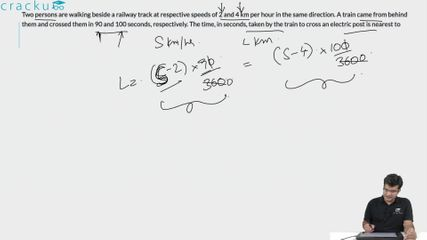Question 21

# Two persons are walking beside a railway track at respective speeds of 2 and 4 km per hour in the same direction. A train came from behind them and crossed them in 90 and 100 seconds, respectively. The time, in seconds, taken by the train to cross an electric post is nearest to

Solution

Let the length of the train be $$l kms$$ and speed be $$s kmph$$. Base on the two scenarios presented, we obtain:

$$\frac{l}{s-2}=\frac{90}{3600}$$....(i) and $$\frac{l}{s-4}=\frac{100}{3600}$$...(ii)

On dividing (ii) by (i) and simplifying we acquire the value of $$s$$ as $$22 kmph$$. Substituting this value in (i), we have $$l=\frac{90}{3600}\times\ 20\ kms$$ {keeping it in km and hours for convenience}

Since we need to find $$\frac{l}{s}$$, let this be equal to $$x$$. Then, $$x\ =\ 90\times\frac{20}{22}\ =81.81\ \approx\ 82\ \sec onds\$$

Hence, Option B is the correct choice.

### View Video Solution